# LIC AAO/SBI PO Prelims Quantitative Aptitude Questions 2019 (Day-02)

Dear Aspirants, Our IBPS Guide team is providing new series of Quantitative Aptitude Questions for LIC AAO/SBI PO 2019 so the aspirants can practice it on a daily basis. These questions are framed by our skilled experts after understanding your needs thoroughly. Aspirants can practice these new series questions daily to familiarize with the exact exam pattern and make your preparation effective.

[WpProQuiz 5473]

### Click Here for SBI PO Pre 2019 High-Quality Mocks Exactly on SBI Standard

Directions (Q. 1 – 2): A number is given in each question with three series I, II and III. Find the series which exactly replaces the question mark with the number and choose the options accordingly.

1) 42

I) 32, 33, ? , 67, 116

II) 84, 84, ? , 14, 3.5

III) 40, ? , 87, 265, 1065

a) Only I

b) Only III

c) Only I and II

d) Only II and III

e) All I, II and III

2) 645

I) 541, 543, 552, 580, ? , 771

II) 212, 568, ? , 835, 879.5, 901.75

III) 1184, 592, ? , 1184, 4736

a) Only I

b) Only II

c) Only III

d) Only I and II

e) Only II and III

Directions (Q. 3 – 5): Find the wrong term in the following number series?

3) 15, 16, 33, 105, 424, 2125

a) 16

b) 33

c) 424

d) 105

e) 2125

4) 3328, 1662, 829, 412.5, 204.75

a) 412.5

b) 3328

c) 204.75

d) 829

e) 1662

5) 786, 843, 908, 1005, 1228

a) 843

b) 1005

c) 1228

d) 786

e) 908

Directions (Q. 6 – 10): Study the following graph carefully and answer the given question.

The following table shows the total number of men and women in a town and the line graph shows the percentage distribution of total number of diabetic men and women in a town over the years.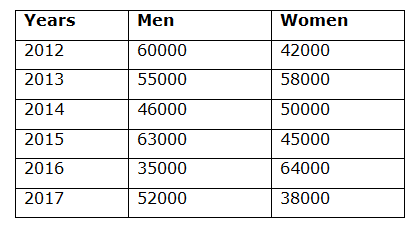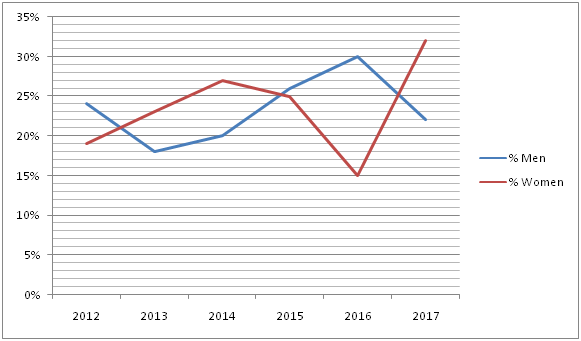6) Find the ratio of total number of diabetic women in the year 2013 and 2015 together to that of total number of diabetic men in the year 2012 and 2017 together?

a) 1372: 1623

b) 557: 619

c) 2459: 2584

d) 23: 62

e) None of these

7) Find the difference between the total number of men to that of women in all the given years together?

a) 14000

b) 26000

c) 8000

d) 32000

e) None of these

8) Total number of diabetic men in the year 2013 and 2015 together is approximately what percentage more/less than total number of diabetic women in the year 2014 and 2017 together?

a) 2 % less

b) 16 % more

c) 16 % less

d) 2 % more

e) 24 % less

9) Find the average number of women in all the given years together?

a) 43000

b) 49500

c) 51500

d) 54000

e) None of these

10) Total number of men in the year 2014 and 2015 together is what percentage of total number of women in the year 2015 and 2016 together?

a) 50 %

b) 75 %

c) 100 %

d) 125 %

e) 150 %

Direction (1-2) :

Series I pattern: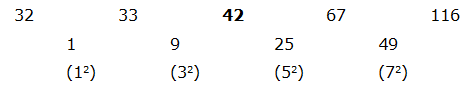The difference is, 12, 32, 52, 72,…

Series II pattern:

84 ÷ 1 = 84

84 ÷ 2 = 42

42 ÷ 3 = 14

14 ÷ 4 = 3.5

Series III pattern:

40*1 + 2 = 42

42*2 + 3 = 87

87*3 + 4 = 265

265*4 + 5 = 1065

Series I pattern: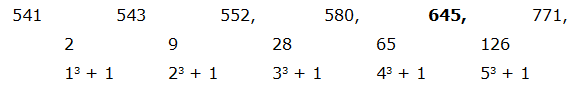The difference is, 13 + 1, 23 + 1, 33 + 1, 43 + 1, 53 + 1,

Series II pattern: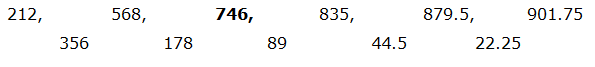The pattern is, the difference between two numbers ÷ 2

Series III pattern:

1184*0.5 = 592

592*1 = 592

592*2 = 1184

1184*4 = 4736

Direction (3-5) :

The correct series is,

15, 16, 34, 105, 424, 2125

The pattern is, *1 + 1, *2 + 2, *3 + 3, *4 + 4, *5 + 5,….

The wrong term is, 33

The correct series is,

3328, 1662, 829, 412.5, 204.25

The pattern is, – 4 ÷ 2

The wrong term is, 204.75

The correct series is,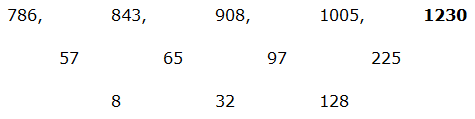The difference of difference is, *4

The wrong term is, 1228

Direction (6-10) :

Total number of diabetic women in the year 2013 and 2015 together

= > 58000*(23/100) + 45000*(25/100)

= > 13340 + 11250 = 24590

Total number of diabetic men in the year 2012 and 2017 together

= > 60000*(24/100) + 52000*(22/100)

= > 14400 + 11440 = 25840

Required ratio = 24590: 25840 = 2459: 2584

The total number of men in all the given years together

= > 60000 + 55000 + 46000 + 63000 + 35000 + 52000 = 311000

The total number of women in all the given years together

= > 42000 + 58000 + 50000 + 45000 + 64000 + 38000 = 297000

Required difference = 311000 – 297000 = 14000

Total number of diabetic men in the year 2013 and 2015 together

= > 55000*(18/100) + 63000*(26/100)

= > 9900 + 16380 = 26280

Total number of diabetic women in the year 2014 and 2017 together

= > 50000*(27/100) + 38000*(32/100)

= > 13500 + 12160 = 25660

Required % = [(26280 – 25660)/25660]*100 = 2.416 % = 2 % more

The total number of women in all the given years together

= > 42000 + 58000 + 50000 + 45000 + 64000 + 38000 = 297000

Required average = 297000/6 = 49500

Total number of men in the year 2014 and 2015 together

= > 46000 + 63000 = 109000

Total number of women in the year 2015 and 2016 together

= > 45000 + 64000 = 109000

Required % = (109000/109000)*100 = 100 %